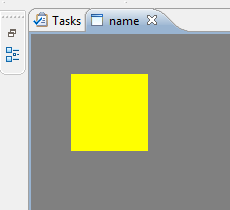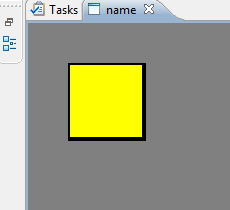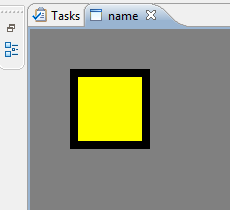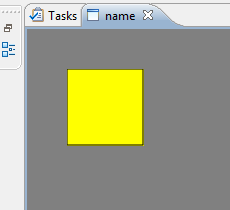# Monthly Archives: November 2012

1 post

## Eclipse Draw2D Border Magic

While using Eclipse Draw2D I stumbled over some quite interesting behaviour on win32 using rectangles and scaling.

We have a scalable layer and added a rectangle. When the layer was actually scaling (so not 1.0) strange artifacts appeared on win32 platforms. Finally it turned out that it was a negative line size with the outline flag set to true. Which renders on Linux as if outline would be set to false. Yet interesting was that setting the lineSize to 0.0 (zero) while keeping the outline flag true causes a hairline to be drawn. So a line which is 1 pixel, independent of the scale factor.

Settings Screenshot
[code language=”Java”]
figure.setOutline ( false );
[/code][code language=”Java”]
figure.setOutline ( true );
[/code]

Which is the same as:
[code language=”Java”]
figure.setOutline ( true );
figure.setLineWidthFloat ( 1.0f );
[/code][code language=”Java”]
figure.setOutline ( true );
figure.setLineWidthFloat ( 0.0f );
[/code]

This is the actual hairline variant.[code language=”Java”]
figure.setOutline ( true );
figure.setLineWidthFloat ( 2.0f );
[/code][code language=”Java”]
figure.setOutline ( true );
figure.setLineWidthFloat ( -1.0f );
[/code]

Note the small artefacts at on the sides. These are invisible if run under Linux.The full code to create the canvas was:

[code language=”Java”]
ScalableLayeredPane pane = new ScalableLayeredPane();

Layer layer = new Layer();
layer.setLayoutManager(new StackLayout());

Figure figure = new Figure ();
figure.setLayoutManager(new XYLayout());
figure.setBackgroundColor(Display.getDefault().getSystemColor(SWT.COLOR_DARK_GRAY));
figure.setOpaque(true);

RectangleFigure r = new RectangleFigure();
r.setBackgroundColor(Display.getDefault().getSystemColor(SWT.COLOR_YELLOW));